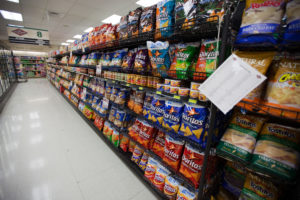## Putting It Together: Real NumbersRemember Jose, the treasurer of the Aspiring Entrepreneurs Club? We left him standing in the grocery store aisle, puzzling over how many bags of chips and packages of cookies to buy for the party he was planning. Let’s review what we know before we apply what you’ve learned to solve the problem:

• Jose has a budget of $76$.
• A bag of chips costs $3$.
• A package of cookies costs $2$.
• A 12-pack of soda costs $5$.
• Jose needs to buy five packs of soda.
• Jose wants to buy two more bags of chips than packages of cookies.

Let’s start by setting up our equation.

We know we’re going to add together three amounts to get the total cost of snacks and drinks for the party: the cost of chips, the cost of cookies, and the cost of drinks.

Next, let’s plug in the numbers that we know. Jose is going to buy five 12-packs of soda, and each of them costs $5$.

So we can write the cost of soda like this:

Cost of soda: $5(5)$ or simply $5(5)$

But what about the costs of chips and cookies? If we let the number of packages of cookies equal $x$, we can write the number of packages of chips as $x+2$. Then we need to multiply by the price of each:

Cost of cookies: $2(x)$ or simply $2x$

Cost of chips: $3(x+2)$ or simply $3(x+2)$

Now we can set up our equation (cost of soda + cookies + chips):

$5(5)+2x+3(x+2)=76$

Let’s use the Distributive Property to remove the parentheses:

$5\cdot 5=25$   and   $3(x+2)=3x+6$

$25+2x+3x+6=76$

Now we can use the Commutative Property of Addition to combine like terms and simplify:

$2x+3x+25+6=76$

$5x+31=76$

With both sides of the expression simplified, we can use the Subtraction Property of Equality. Subtract $31$ from both sides

$5x+31\color{red}{-31}=76\color{red}{-31}$

$5x=45$

Finally, we can use the Division Property of Equality to divide both sides by $5$ and solve for $x$:

${\Large\frac{5x}{5}}={\Large\frac{45}{5}}$

$x=9$

Earlier, we defined $x$ as the number of cookie packages. Based on our calculations, Jose can buy $9$ packages of cookies. Remember that he wants to buy two more bags of chips than packages of cookies, so that equals $x+2=9+2=11$. Jose can buy $11$ bags of chips.

Thanks to Jose, the refreshments at the Aspiring Entrepreneurs Club’s party will be plentiful and within budget! Are you excited to try applying some math when planning your next party?

## Contribute!

Did you have an idea for improving this content? We’d love your input.